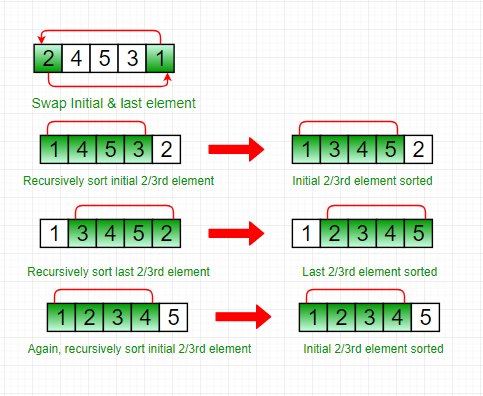# Stooge Sort

• Difficulty Level : Hard
• Last Updated : 18 May, 2022

Stooge Sort is a recursive sorting algorithm. It is not much efficient but interesting sorting algorithm. It generally divides the array into two overlapping parts (2/3 each). After that it performs sorting in first 2/3 part and then it performs sorting in last 2/3 part. And then, sorting is done on first 2/3 part to ensure that the array is sorted.

The key idea is that sorting the overlapping part twice exchanges the elements between the other two sections accordingly.

Approach:

Step 1: If value at index 0 is greater than value at last index, swap them.
Step 2: Recursively,

• Stooge sort the initial 2/3rd of the array.
• Stooge sort the last 2/3rd of the array.
• Stooge sort the initial 2/3rd again to confirm.

NOTE: Always take the ceil of ((2/3)*N) for selecting elements.

Illustration:

Lets consider an example: arr[] = {2, 4, 5, 3, 1}

• Step1: Initially, First and last elements are compared and if last is greater than first then they are swapped.
• Step2: Now, recursively sort initial 2/3rd of the elements as shown below:
• Step3: Then, recursively sort last 2/3rd of the elements, as shown below:
• Step4: Again, sort the initial 2/3rd of the elements to confirm final data is sorted.
• Resulted array:Below is the implementation for the above approach:

## C++

 `// C++ code to implement stooge sort``#include ``using` `namespace` `std;` `// Function to implement stooge sort``void` `stoogesort(``int` `arr[], ``int` `l, ``int` `h)``{``    ``if` `(l >= h)``        ``return``;` `    ``// If first element is smaller than last,``    ``// swap them``    ``if` `(arr[l] > arr[h])``        ``swap(arr[l], arr[h]);` `    ``// If there are more than 2 elements in``    ``// the array``    ``if` `(h - l + 1 > 2) {``        ``int` `t = (h - l + 1) / 3;` `        ``// Recursively sort first 2/3 elements``        ``stoogesort(arr, l, h - t);` `        ``// Recursively sort last 2/3 elements``        ``stoogesort(arr, l + t, h);` `        ``// Recursively sort first 2/3 elements``        ``// again to confirm``        ``stoogesort(arr, l, h - t);``    ``}``}` `// Driver Code``int` `main()``{``    ``int` `arr[] = { 2, 4, 5, 3, 1 };``    ``int` `n = ``sizeof``(arr) / ``sizeof``(arr);` `    ``// Calling Stooge Sort function to sort``    ``// the array``    ``stoogesort(arr, 0, n - 1);` `    ``// Display the sorted array``    ``for` `(``int` `i = 0; i < n; i++)``        ``cout << arr[i] << ``" "``;` `    ``return` `0;``}`

## Java

 `// Java program to implement stooge sort``import` `java.io.*;` `public` `class` `stooge {``    ``// Function to implement stooge sort``    ``static` `void` `stoogesort(``int` `arr[], ``int` `l, ``int` `h)``    ``{``        ``if` `(l >= h)``            ``return``;` `        ``// If first element is smaller``        ``// than last, swap them``        ``if` `(arr[l] > arr[h]) {``            ``int` `t = arr[l];``            ``arr[l] = arr[h];``            ``arr[h] = t;``        ``}` `        ``// If there are more than 2 elements in``        ``// the array``        ``if` `(h - l + ``1` `> ``2``) {``            ``int` `t = (h - l + ``1``) / ``3``;` `            ``// Recursively sort first 2/3 elements``            ``stoogesort(arr, l, h - t);` `            ``// Recursively sort last 2/3 elements``            ``stoogesort(arr, l + t, h);` `            ``// Recursively sort first 2/3 elements``            ``// again to confirm``            ``stoogesort(arr, l, h - t);``        ``}``    ``}` `    ``// Driver Code``    ``public` `static` `void` `main(String args[])``    ``{``        ``int` `arr[] = { ``2``, ``4``, ``5``, ``3``, ``1` `};``        ``int` `n = arr.length;` `        ``stoogesort(arr, ``0``, n - ``1``);` `        ``for` `(``int` `i = ``0``; i < n; i++)``            ``System.out.print(arr[i] + ``" "``);``    ``}``}``// Code Contributed by Mohit Gupta_OMG <(0_o)>`

## Python3

 `# Python program to implement stooge sort` `def` `stoogesort(arr, l, h):``    ``if` `l >``=` `h:``        ``return`` ` `    ``# If first element is smaller``    ``# than last, swap them``    ``if` `arr[l]>arr[h]:``        ``t ``=` `arr[l]``        ``arr[l] ``=` `arr[h]``        ``arr[h] ``=` `t`` ` `    ``# If there are more than 2 elements in``    ``# the array``    ``if` `h``-``l ``+` `1` `> ``2``:``        ``t ``=` `(``int``)((h``-``l ``+` `1``)``/``3``)`` ` `        ``# Recursively sort first 2 / 3 elements``        ``stoogesort(arr, l, (h``-``t))`` ` `        ``# Recursively sort last 2 / 3 elements``        ``stoogesort(arr, l ``+` `t, (h))`` ` `        ``# Recursively sort first 2 / 3 elements``        ``# again to confirm``        ``stoogesort(arr, l, (h``-``t))`` `  `# deriver``arr ``=` `[``2``, ``4``, ``5``, ``3``, ``1``]``n ``=` `len``(arr)` `stoogesort(arr, ``0``, n``-``1``)`` ` `for` `i ``in` `range``(``0``, n):``    ``print``(arr[i], end ``=` `' '``)` `# Code Contributed by Mohit Gupta_OMG <(0_o)>`

## C#

 `// C# program to implement stooge sort``using` `System;` `class` `GFG {``    ` `    ``// Function to implement stooge sort``    ``static` `void` `stoogesort(``int``[] arr,``                            ``int` `l, ``int` `h)``    ``{``        ``if` `(l >= h)``            ``return``;` `        ``// If first element is smaller``        ``// than last, swap them``        ``if` `(arr[l] > arr[h]) {``            ``int` `t = arr[l];``            ``arr[l] = arr[h];``            ``arr[h] = t;``        ``}` `        ``// If there are more than 2``        ``// elements in the array``        ``if` `(h - l + 1 > 2) {``            ``int` `t = (h - l + 1) / 3;` `            ``// Recursively sort first``            ``// 2/3 elements``            ``stoogesort(arr, l, h - t);` `            ``// Recursively sort last``            ``// 2/3 elements``            ``stoogesort(arr, l + t, h);` `            ``// Recursively sort first``            ``// 2/3 elements again to``            ``// confirm``            ``stoogesort(arr, l, h - t);``        ``}``    ``}` `    ``// Driver Code``    ``public` `static` `void` `Main()``    ``{``        ``int``[] arr = { 2, 4, 5, 3, 1 };``        ``int` `n = arr.Length;` `        ``// Calling Stooge Sort function``        ``// to sort the array``        ``stoogesort(arr, 0, n - 1);` `        ``// Display the sorted array``        ``for` `(``int` `i = 0; i < n; i++)``            ``Console.Write(arr[i] + ``" "``);``    ``}``}` `// This code is contributed by Sam007.`

## Javascript

 ``
Output
`1 2 3 4 5 `

The running time complexity of stooge sort can be written as,
T(n) = 3T(3n/2) + ?(1)

Solution of above recurrence is O(n(log3/log1.5)) = O(n2.709), hence it is slower than even bubble sort(n^2).
This article is contributed by DANISH KALEEM. If you like GeeksforGeeks and would like to contribute, you can also write an article using write.geeksforgeeks.org or mail your article to review-team@geeksforgeeks.org. See your article appearing on the GeeksforGeeks main page and help other Geeks.

My Personal Notes arrow_drop_up